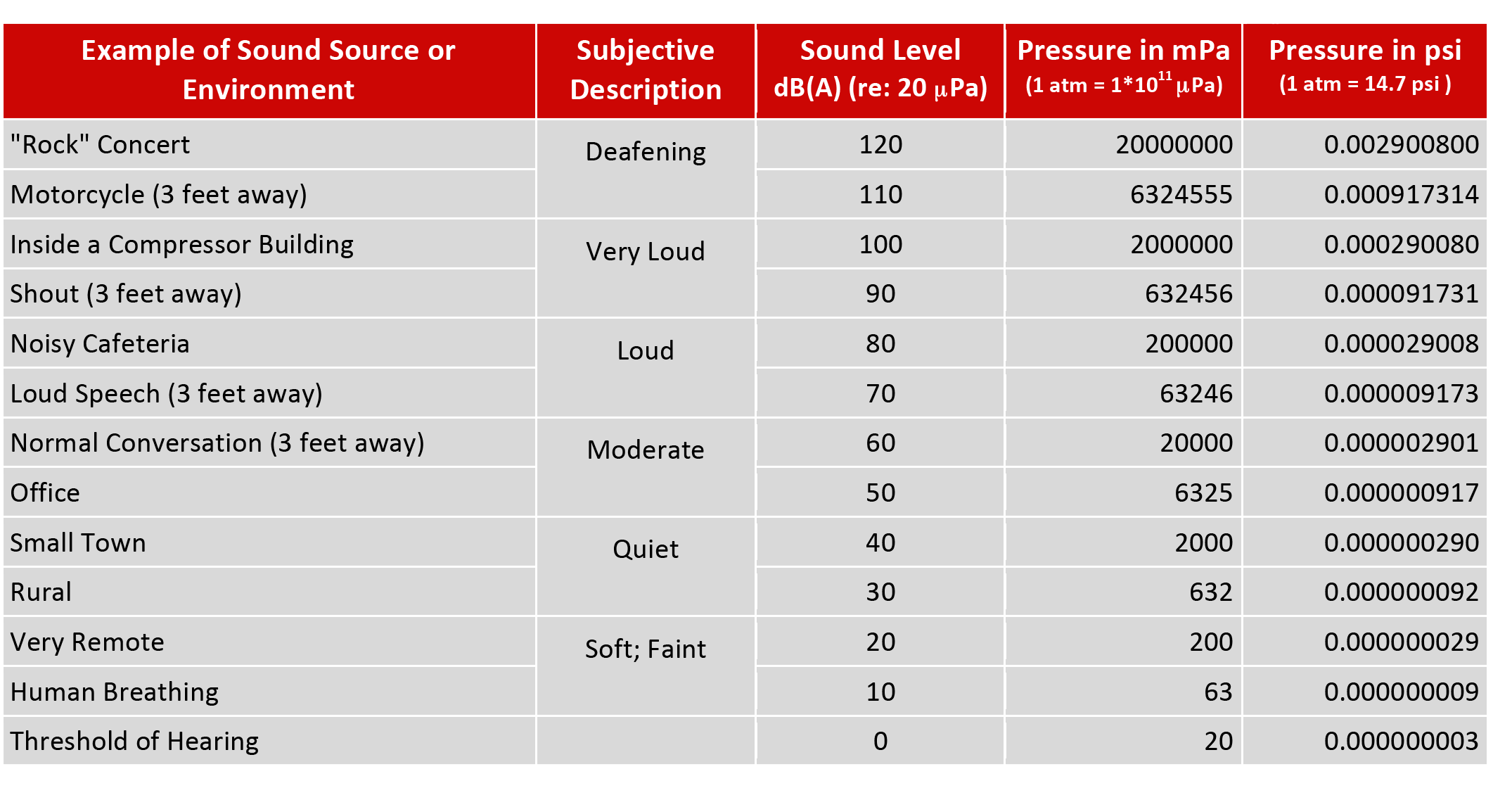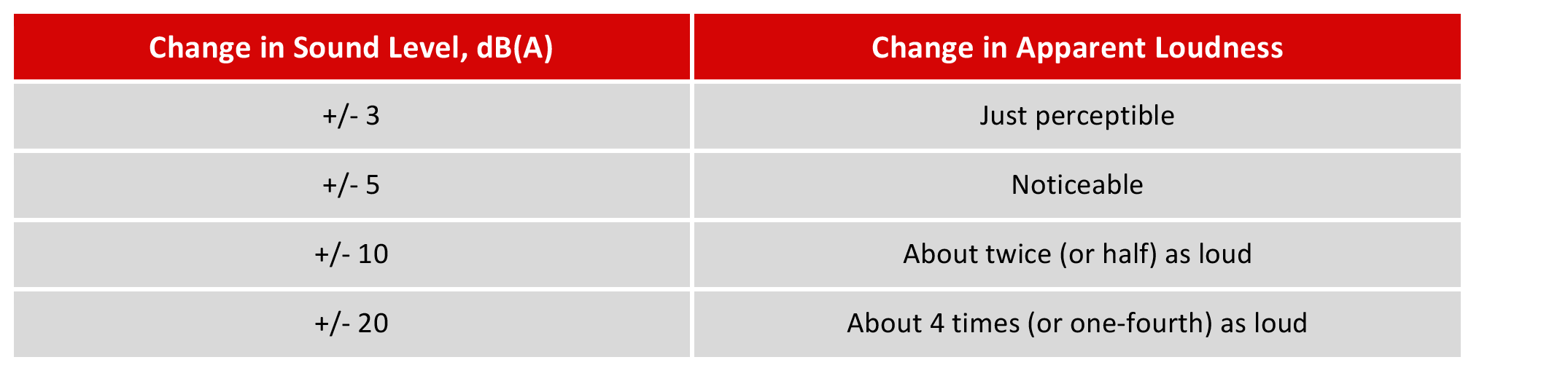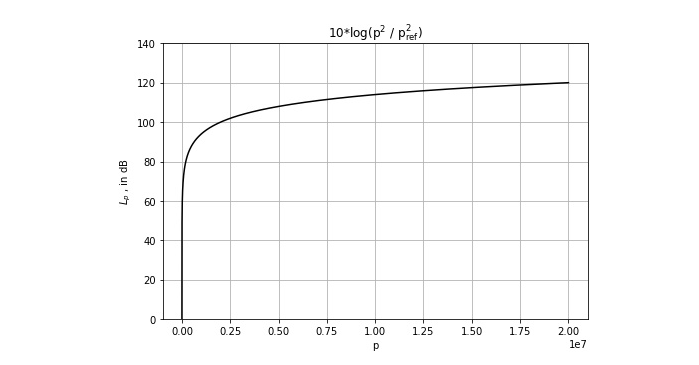United Steel Structures - The USSI Blog - Acoustical Rules of Thumb and Demystifying the Decibel

# Acoustical Rules of Thumb and Demystifying the Decibel

Decibels can cause confusion. For example, if a bird is singing its song at a sound level of 60 dB(A) and another one joins in at the same volume, then the total sound level is not 120 dB(A), it is actually 63 dB(A). Also, there is a general lack of familiarity with how decibel sound levels are related to how loud a sound is perceived. Lastly, if you attempt to seek out a definition of a decibel, you will likely find one that is written for or by an engineer, which may or may not be helpful. In this post, I will start with real-world examples of decibels in action, provide some context and rules of thumb, and work up to the formal definition, which is not really necessary in order to gain a working knowledge of decibel sound levels.

###### Three things to know:

• 1. How loud are sounds?
• 2. How does one decibel value compare to another?
• 3. What is a decibel, often seen as "dB" or "dB(A)?"
###### 1. Sound Level Examples

The first thing that should be appreciated is that we humans can hear a remarkably huge range of sounds. The vast extent between the quietest sound we can hear and the loudest sound we can withstand is truly amazing.

The following table lists sound sources and sound environments with which you may be familiar. The next column shows a subjective description of how that sound would be perceived by a typical listener. Next to that is a typical decibel value (A-weighted sound level, abbreviated dB(A)) of that sound or environment. Focusing on the first three columns, we can see that at 0 decibels, we are just beginning to be able to hear a sound and at 120 decibels or more, sound begins to be physically painful.

Two good benchmarks to remember are that at arms' length away:

• 1) a person speaking in a normal conversational voice corresponds to a sound level of about 60 dB(A) and
• 2) when that person shouts, the level is about 90 dB(A).

I should note that the decibel values represented below are typical values for generic sources or environments. One person's voice level may be a good bit louder than another and one small town may quieter than another.1 atm = 14.7 psi = 1*1011 μPa = 1*105 Pa (atm is "atmospheres", psi is "pounds-per-square-inch", and Pa is "Pascals")

Now, let us look at the last two columns, which show the same quantity (pressure), but expressed in different units. First, notice how much the numbers increase from the bottom to the top and in particular, how large the pressure is of a deafening sound versus the faintest sound we can hear. Such a vast range of numbers makes it difficult to conveniently talk quantitatively about all the sounds within the human hearing experience, so converting these values to something more manageable is of great benefit. This is the basic reason why we prefer to use decibels to discuss sound levels. As we soon will see, the decibel is based on the logarithm function, which is a mathematical way of taking increasingly large numbers and compressing them to a more manageable scale.

The columns labeled "Pressure" represent the acoustic pressure, or gauge pressure if you will, of the respective decibel level. The acoustic pressure fluctuates in addition to atmospheric pressure. Acoustic pressures are very, very small compared to everyday pressures such as the 32 psi you might put in your tires, or 8 psi you might put in a basketball. Atmospheric pressure (1 atm) is roughly 5000 times the acoustic pressure of a "deafening" sound. The fact that acoustic pressures are so small is another reason why decibels make sense, because it allows us to state the acoustic pressure relative to a reference pressure and not worry about the specific pressure unit.

###### 2. Comparing Decibels; Rules of Thumb

When comparing decibel values, it is important to keep in mind how decibels combine. Consider the following two points:

• The combination of a sound having a level of 50 dB(A), and a second sound having an equal sound level of 50 dB(A) is 53 dB(A). In shorthand, 50 dB(A) "plus" 50 dB(A) is 53 dB(A). Hence,
Two equal sound levels combine to be 3 decibels greater than the individual.
• The combination of a sound having a level of 60 dB(A), and a second sound having a sound level of 50 dB(A) is 60 dB(A). In shorthand, 60 dB(A) "plus" 50 dB(A) is 60 dB(A). Hence,
When two sound levels differ by 10 decibels or more, when combined, the lesser level can be ignored.

When comparing the perceived relative loudness of a change in sound level, consider the following table. Loudness is the term we use for how we rank our perception of various sound levels. Loudness is like the volume of the television or media player.For example, if a sound level increases from 40 dB(A) to 50 dB(A) – an increase of +10 dB(A) – it would be perceived as being twice as loud as it was originally. If the level increased to 60 dB(A) it would then be perceived as being four times as loud as it was when it was 40 dB(A). Hence, the following two rules of thumb:

• A sound needs to increase by more than 3 decibels for it to be noticeable.
• For every increase of 10 decibels, the apparent sound loudness doubles.
###### 3. What is a Decibel?

At last, we define a decibel, for which the symbol is “dB”: A decibel is ten times the logarithm (base 10) of the ratio of a power-type quantity to a reference value of the same unit. (Now you might see why I didn't lead with that.) Acoustic power is proportional to the square of the (acoustic) pressure, so we define the decibel sound pressure level, Lp, aswhere pref^2 is the reference pressure of 20 μPa, the lowest audible acoustic pressure (the threshold of hearing). The figure below shows the graph of Lp in dB for the pressure values shown above in the table of example sound levels. Note that while the pressure ranges from 20 to 20,000,000 μPa, (six orders of magnitude), the corresponding decibel values range from 0 to 120 dB; reasonable numbers for everyday purposes.###### Summary

In order to talk about how loud a sound is we begin with the pressure of the sound, which is a physical quantity that can be measured and quantified. We then convert the pressure into a decibel sound level in order to be able to accommodate the vast range of pressures audible to humans. Because decibels can be confusing to work with, the following rules of thumb are helpful to remember:

• Normal conversation at arms' length is about 60 dB(A)
• A loud shout at arms' length is about 90 dB(A)
• Two equal sound levels combine to be 3 decibels greater than the individual.
• When two sound levels differ by 10 decibels or more, when combined, the lesser level can be ignored.
• A sound needs to increase by at least 3 decibels for it to be noticeable.
• For every increase of 10 decibels, the apparent sound loudness doubles.About the Author - Tim Simmons, Ph.D. - USSI Director of Industrial Acoustics
Dr. Tim Simmons manages USSI's Industrial Acoustics Department. Tim comes to USSI with a wealth of Acoustical and Noise Control knowledge and real-world experience. Tim holds a Ph.D. in Physics from the University of Mississippi and a B.S. in Engineering Physics from the University of Tennessee.
Member INCE, ASA, ASME